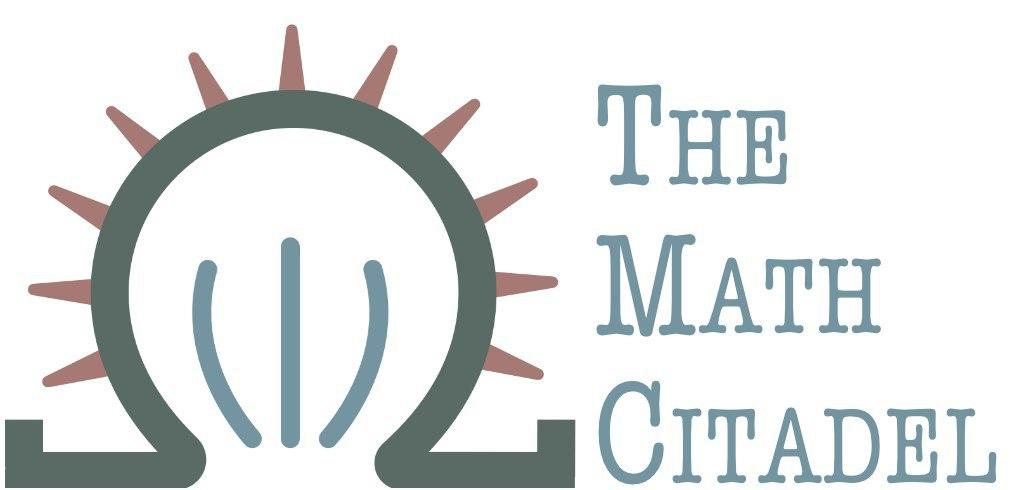## Applications of Reflections: Taking a Group to its Abelian Form

#### R. Traylor

This article looks at the definition of the categorical concept of a reflection and gives a clear application with full construction. For some previous discussion of categorical concepts and more basic definitions, see the joint article by R. Traylor and V. Fadeev.

Definition (Subcategory):

A category $\mathbf{A}$ is a subcategory of a category $\mathbf{B}$ if

1. $\mathrm{Ob}(\mathbf{A}) \subseteq \mathrm{Ob}(\mathbf{B})$
2. For each $A, A' \in \mathrm{Ob}(\mathbf{A})$, $\mathrm{hom}_{\mathbf{A}}(A,A') \subseteq \mathrm{hom}_{\mathbf{B}}(A,A')$.
3. For each $A \in \mathrm{Ob}(\mathbf{A})$, the $\mathbf{B}-$identity on $A$ is the same as the $\mathbf{A}-$identity on $A$.
(4) composition in $\mathbf{A}$ is the restriction of composition in $\mathbf{B}$ to the morphisms of $\mathbf{A}$
Adámek et al, Abstract and Concrete Categories (1990)

Point by point, we'll pick the definition apart. The first part is pretty clear. The collection of objects in a subcategory is contained in the collection of objects in its "parent". The second criterion says that the set of morphisms from one object $A$ to another object $A'$ inside the littler category $\mathbf{A}$ should be a subset of all the morphisms from the same $A$ to the same $A'$, but inside $\mathbf{B}$. That is, there are morphisms from $A \to A'$ in $\mathbf{B}$ that won't live in the subcategory $\mathbf{A}$.

The third criterion just states that the identity morphisms on objects should match in both categories. The final criterion tells us that composition inside the subcategory $\mathbf{A}$ only "works" on the morphisms inside $\mathbf{A}$, but is otherwise the same composition as in $\mathbf{B}$. We just only perform compositions on morphisms in $\mathbf{A}$ when we're in $\mathbf{A}$.

We now define a reflection.

Definition ($A-$ reflection)

Let $\mathbf{A}$ be a subcategory of $\mathbf{B}$, and let $B \in \mathrm{Ob}(\mathbf{B})$.

(1) An $\mathbf{A}-$ reflection for $B$ is a morphism $B \xrightarrow{r} A$ from $B$ to $A \in \mathrm{Ob}(\mathbf{A})$ such that for any morphism $B \xrightarrow{f} A'$ from $B$ to $A' \in \mathrm{Ob}(\mathbf{A})$ there exists a unique $f': A \to A'$ such that $f = f' \circ r$.
(2) $\mathbf{A}$ is a reflective subcategory for $\mathbf{B}$ if each $\mathbf{B}-$ object has an $\mathbf{A}-$reflection.

Currently, this definition seems a bit abstract. The following sections will illustrate concrete examples of reflections to understand this definition better.

## Taking a Group to Its Abelian Form

### The Category $\mathbf{Grp}$

For this example, we'll be working with the category of groups, $\mathbf{Grp}$. The objects in this category are groups, and the morphisms are group homomorphisms. Some elements of this category are:

• $(\mathbb{R}, +) \xrightarrow{\phi} (\mathbb{R}^{+}, \cdot)$, $\phi(x) = e^{x}$. The real numbers under addition and the positive real numbers under multiplication are both groups, so they're objects in this category. $\phi$ here is a group homomorphism (actually an isomorphism), so it's a morphism in $\mathbf{Grp}$.
• The dihedral group $D_{6}$, and the permutation group $S_{3}$ are also objects in this class. Recall that the dihedral group $D_{6}$ is commonly visualized using the symmetries of an equilateral triangle. (There are 6 symmetry elements. Three reflections, with the reflection lines passing through each of the three vertices, two rotations of angle $2k\pi/3, k=1,2$, and an identity element that does nothing.), More commonly, the dihedral group is given using the following presentation: $$D_{6} = \langle r,s : r^{3} = s^{2} = 1, sr = r^{-1}s\rangle$$ Here, we see that $D_{6}$ is generated by two elements $r$ and $s$ ($r$ is the rotation by $2\pi/3$, and $s$ is one of the reflection lines). $S_{3}$ is the set of permutations on 3 elements-- all permutations of the integers $\{1,2,3\}$. Both of these are groups, and both have 6 elements. If we define $$\phi: \begin{cases} r \to (123) \\ s \to (12)\end{cases}$$ then $\phi$ is a group homomorphism that takes $D_{6}$ to $S_{3}$, and is also thus a morphism in $\mathbf{Grp}$.
• As one last example, let $\mathrm{GL}_{2}(\mathbb{R})$ be the general linear group of degree $2$. This is the group of invertible $2\times 2$ matrices with real entries. Then we can create a group homomorphism $\phi: D_{6} \to \mathrm{GL}_{2}(\mathbb{R})$ by letting $$\phi(r) = \begin{bmatrix}\cos(\theta) & -\sin(\theta)\\ \sin(\theta) & \cos(\theta)\end{bmatrix} \qquad \phi(s) = \begin{bmatrix} 0 & 1\\ 1 & 0 \end{bmatrix}$$ so this $\phi$ is also a morphism in $\mathbf{Grp}$.

### The Category $\mathbf{Ab}$

A subcategory of $\mathbf{Grp}$ is $\mathbf{Ab}$, the category of abelian groups. Morphisms are again group homomorphisms, but this time we are only looking at morphisms between abelian groups. Some examples:

1. $(\mathbb{R}, +) \xrightarrow{\phi} (\mathbb{R}^{+}, \cdot)$, $\phi(x) = e^{x}$. The real numbers under addition, and the positive real numbers under multiplication are abelian groups, so they're in the subcategory $\mathbf{Ab}$.
2. $(\mathbb{Z}_{2} \times \mathbb{Z}_{2}, +)$ and $(\mathbb{Z}_{4}, +)$ are also abelian groups. We can define a group homomorphism $\psi: \mathbb{Z}_{2} \times \mathbb{Z}_{2} \to \mathbb{Z}_{4}$ by $$\psi: \begin{cases} (0,0) \to 0 \\ (1,0) \to 1\\ (0,1) \to 2 \\ (1,1) \to 3\end{cases},$$ so $\psi$ is in the collection of morphisms in $\mathbf{Ab}$. Of course, it's also in the morphisms of $\mathbf{Grp}$ as well.
3. $D_{6}$ is not commutative, so it's not an object in $\mathbf{Ab}$.

## Conclusion

Reflections can perform many different actions to take objects in one category to objects in a subcategory. We focused on reflections making things "abelian" in a nice way, which helped reveal some structures that would have otherwise been hidden.